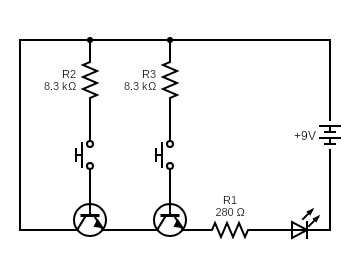# Logic gate resistance problems

Here's an AND gate I built for practice and it's working with one quirk. Whenever I press the button closest to the LED by itself, the LED dimly lights up. I think there's enough voltage going through the transistor base/emitter to power the LED. How can I prevent that from happening? If it matters I have my calculations below.R1 Resistance

Vin = 9V
LED = 3V drop / .02A
Transistor 1 = .2V drop
Transistor 2 = .2V drop

R1 = (9V-3V-0.2V-0.2V)/.02A = 280 Ohms

Transistor Base Current

IC = .02A
HFE(MIN) = 200
Overdrive factor = 10

IB = .02A/200(10) = .001A

R2 and R3 Resistance

VBE(sat) = 0.7

R = (9V-0.7V)/.001A = 8300 OhmsIncrease the base current by reducing the base resistor.

Joined February 12, 2018      696
Monday at 02:11 PMHi,When driving an NPN bipolar transistor (emitter to GND) with a logic gate output, most logic families have a low enough logic 0 output voltage to turn off the transistor without a pull-down resistor to GND. If you want to add one, put it between the base and GND, not the gate output and GND. Thanks!

Joined August 13, 2021      3
Friday at 04:51 PM